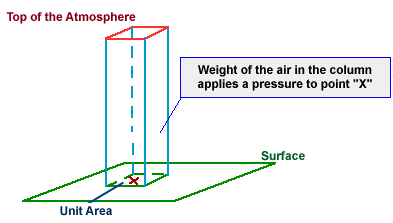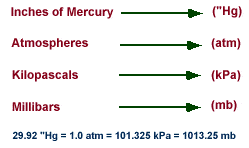# How much atmospheric pressure is exerted on the human body?

I understand that this will vary from human to human, but how much weight is exerted, in total, on the average adult human body. It would be very much appreciated if the calculations can be shown. Thanks!

The average pressure exerted on the human body by the atmosphere is the average local atmospheric pressure. At sea level this is about 101kPa.

The average weight exerted on the human body by the atmosphere is the weight of the atmosphere above an individual. This is equal to the local pressure times the surface area facing up. I have seen the surface area on the top of a human head and shoulders estimated at .1 m2.

This gives a weight of 10.1 kN, or just a little over 1.1 ton.

sophiecentaur
Gold Member
how much weight is exerted, in total, on the average adult human body.
I suspect that you are looking for an answer to a question that has been given you or to settle an argument (?). The essence of what's going on is not really explainable in the terms of your question. It's really important to distinguish between Force (eg. weight) and Pressure (e.g. Newtons per square metre))
The 'weight force' of the Atmosphere on an object, acts inwards in all directions and is described as Atmospheric Pressure (which we all know). Our bodies are doing nothing particularly clever by balancing the inward pressure from the atmosphere with the internal outward pressure; everything does this. We have no strength to fight against atmospheric pressure so our internal pressure is basically the same as outside. It's the same for a mouse or an elephant; the pressures are balanced even though the total squashing forces are very different. Submarines, an exception, have rigid hulls which keep their internal pressure much lower than the outside water pressure.
The ambient pressure is 'right' for us (all animals) to breathe in enough Oxygen to keep alive and to stop us losing too much body fluid by evaporation etc. etc. But it's all to do with Pressure and not Weight of Atmosphere. In a space ship, the pressure is maintained with Pumps and doesn't involve the 'weight of a column of air'.

The net effect of the atmospheric pressure this is a very small upward Force. We experience Upthrust because we are immersed (Archimedes Principle) in a fluid (the air) in the same way that we experience (much more) upthrust when we are in water. The weight of displaced air will be something in the region of
-Volume displaced X density X g
= -80Λ-3 X 1.2 X 9.8N
=about -0.9N, which is the Upthrust Force. (Equivalent to the weight of 90g of water)
which would be measurable - but not easily because we would need to measure our weight also in a vacuum and compare the two values.

I understand that this will vary from human to human, but how much weight is exerted, in total, on the average adult human body. It would be very much appreciated if the calculations can be shown. Thanks!
I guess what you really want to know is the total atmospheric force exerted on the human body. The average human body surface area is 1.73 m2 and the standard atmospheric pressure is 101,325 N/m2. Hence the total atmospheric force exerted on the average human body would be 1.73 m2 x 101,325 N/m2 = 175,300 N (approx.).

Assuming standard gravitational acceleration as 9.80665 m/s2, this would be equivalent to 175,300/9.80665 kgf = 17,900 kgf.

That's a lot of force acting on the body but it's all counterbalanced by the internal pressure of the body and so you won't feel it at all. Hope this is the real answer you're looking for.

Keith_McClary
Gold Member
The average human body surface area is 1.73 m2
I was going to give a spherical cow answer.

Chestermiller
Mentor
I guess what you really want to know is the total atmospheric force exerted on the human body. The average human body surface area is 1.73 m2 and the standard atmospheric pressure is 101,325 N/m2. Hence the total atmospheric force exerted on the average human body would be 1.73 m2 x 101,325 N/m2 = 175,300 N (approx.).

Assuming standard gravitational acceleration as 9.80665 m/s2, this would be equivalent to 175,300/9.80665 kgf = 17,900 kgf.

That's a lot of force acting on the body but it's all counterbalanced by the internal pressure of the body and so you won't feel it at all. Hope this is the real answer you're looking for.
This is not correct, because the pressure force on a surface is normal to the surface, and, these normal forces on the body must be added vectorially. If you do this correctly, as was done by @mfig, you get the answer he gave.

•256bits and sophiecentaur
This is not correct, because the pressure force on a surface is normal to the surface, and, these normal forces on the body must be added vectorially. If you do this correctly, as was done by @mfig, you get the answer he gave.
Sorry, have to disagree with you. Don't forget, the atmospheric pressure acts upwards as well via all the downward facing surfaces and the NET force would be zero. However, the pressures at the top of the body are slightly lower than at the bottom due to the weight of the air resulting in a small upward force (0.9N) as calculated by sophiecentaur.

But I suspect that Hunter1234 is more interested in the total force that would be crushing the human body if it had been hollow and filled with nothing, ie a vacuum, akin to the submarine deep under the ocean. That was what I was trying to calculate for him.

So I guess the answer really depends on what Hunter1234 is actually asking.

Don't forget, I'm not Bill Nye.

Chestermiller
Mentor
Sorry, have to disagree with you. Don't forget, the atmospheric pressure acts upwards as well via all the downward facing surfaces and the NET force would be zero. However, the pressures at the top of the body are slightly lower than at the bottom due to the weight of the air resulting in a small upward force (0.9N) as calculated by sophiecentaur.

But I suspect that Hunter1234 is more interested in the total force that would be crushing the human body if it had been hollow and filled with nothing, ie a vacuum, akin to the submarine deep under the ocean. That was what I was trying to calculate for him.

So I guess the answer really depends on what Hunter1234 is actually asking.

Don't forget, I'm not Bill Nye.
I respectfully disagree. The forces have to be added vectorially, in my humble judgment. I guess we'll just have to agree to disagree.

sophiecentaur
Gold Member
But I suspect that Hunter1234 is more interested in the total force that would be crushing the human body if it had been hollow and filled with nothing, ie a vacuum, akin to the submarine deep under the ocean. That was what I was trying to calculate for him.
I suspect that you are right about what Hunter 'thinks' he wants to know. But the Force is really of no consequence unless we want to know about bouyancy. The effects of pressure on the human body are not so much Mechanical but Chemical. A human can 'breathe' air in and out at more or less any ambient pressure. The problem arises when the partial pressures of gases in the air (and also within the body) are 'inappropriate' for respiration and the rates of gaseous exchange do not sustain life. (That's why saturation divers use special mixes of gases.)
The Force involved is little more than a naive concept that is brought into things by non-scientists because the 'numbers' look impressive.

jbriggs444
Homework Helper
I guess what you really want to know is the total atmospheric force exerted on the human body. The average human body surface area is 1.73 m2 and the standard atmospheric pressure is 101,325 N/m2. Hence the total atmospheric force exerted on the average human body would be 1.73 m2 x 101,325 N/m2 = 175,300 N (approx.).

Assuming standard gravitational acceleration as 9.80665 m/s2, this would be equivalent to 175,300/9.80665 kgf = 17,900 kgf.

That's a lot of force acting on the body but it's all counterbalanced by the internal pressure of the body and so you won't feel it at all. Hope this is the real answer you're looking for.
The lungs have a lot of surface area. Why not count that? Since the question is nonsensical, the answer should be as well.

•phinds, Bystander and russ_watters
256bits
Gold Member
But I suspect that Hunter1234 is more interested in the total force
If you squeeze an object between your hands, do you add the 10N from the left hand with the 10N from the right hand to get 20N of total force?

•nasu and Chestermiller
Now what would this be for the same human who (unwisely) stepped out of his space capsule unprepared on the surface of Venus?

sophiecentaur
Gold Member
Now what would this be for the same human who (unwisely) stepped out of his space capsule unprepared on the surface of Venus?
Chemical or Thermal effects would be felt first. Secondly, the different pressure could be 'noticeable' but I doubt it.

I understand that this will vary from human to human, but how much weight is exerted, in total, on the average adult human body. It would be very much appreciated if the calculations can be shown. Thanks!
Atmospheric Pressureforce exerted by the weight of the air
Atmospheric pressure is defined as the force per unit area exerted against a surface by the weight of the air above that surface. In the diagram below, the pressure at point "X" increases as the weight of the air above it increases. The same can be said about decreasing pressure, where the pressure at point "X" decreases if the weight of the air above it also decreases.Thinking in terms of air molecules, if the number of air molecules above a surface increases, there are more molecules to exert a force on that surface and consequently, the pressure increases. The opposite is also true, where a reduction in the number of air molecules above a surface will result in a decrease in pressure. Atmospheric pressure is measured with an instrument called a "barometer", which is why atmospheric pressure is also referred to as barometric pressure.In aviation and television weather reports, pressure is given in inches of mercury ("Hg), while meteorologists use millibars (mb), the unit of pressure found on weather maps.

As an example, consider a "unit area" of 1 square inch. At sea level, the weight of the air above this unit area would (on average) weigh 14.7 pounds! That means pressure applied by this air on the unit area would be 14.7 pounds per square inch. Meteorologists use a metric unit for pressure called a millibar and the average pressure at sea level is 1013.25 millibars.
Ref;
http://ww2010.atmos.uiuc.edu/(Gh)/guides/mtr/fw/prs/def.rxml

•Delta2
russ_watters
Mentor
This thread is 5 years old...

•sophiecentaur
Delta2
Homework Helper
Gold Member
This thread is 5 years old...
I don't see what's the problem with that really. Chestermiller and the others are still around and can answer if they find the new reply interesting. The nice thing with electronic threads is that there is no paper yellowed out, no ink worn out, the thread appears as good as new.

Staff Emeritus
But the atmosphere is much the same.••